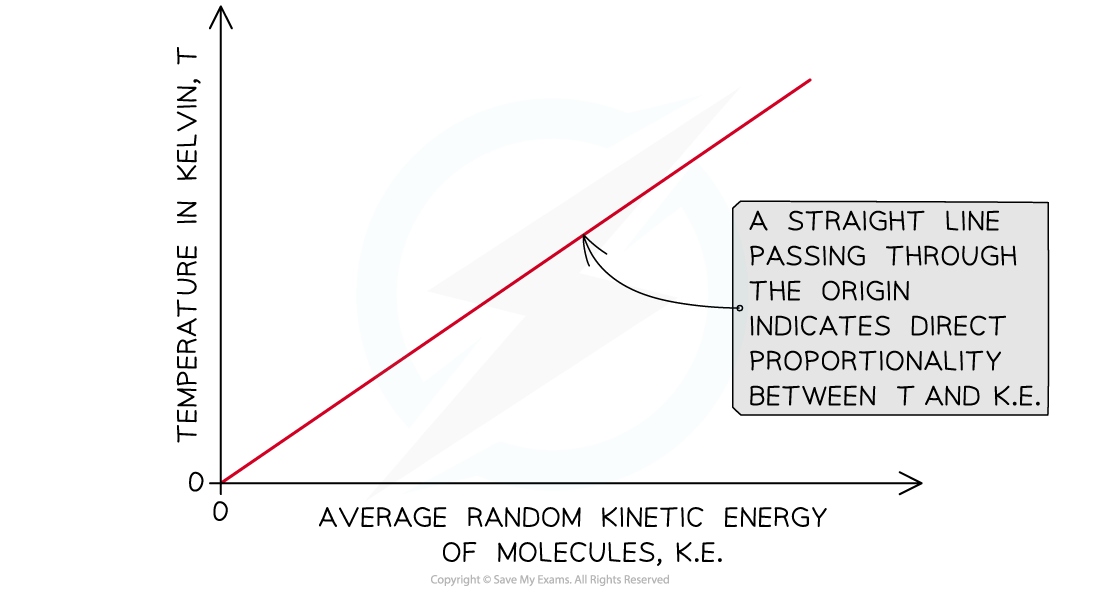# IB DP Physics: SL复习笔记3.1.2 Temperature

### Temperature

• Temperature is a measure of how hot or cold objects are
• Temperature also determines the direction in which thermal energy will flow between two objects (or between an object and its surroundings)
• When thermal energy is exchanged, the objects (or systems) involved are said to have a thermal interaction
• The thermal energy exchanged during a thermal interaction is referred to as heat
• During a thermal interaction:
• Thermal energy always flows from the hotter object to the colder object
• The energy transfer continues until the two objects are in thermal equilibrium (i.e. they both have the same temperature)
• Thermal energy can be transferred via conduction, convection or radiation
• Temperature is a scalar quantity and it is measured using a thermometer
• It is measured in degrees Celsius (°C) or kelvin (K)
• The kelvin is the SI base unit for temperature
• The temperature of an object is a macroscopic measure of the average kinetic energy of the particles (atoms or molecules) that make up the object

#### Absolute temperature

• Absolute temperature is temperature measured in kelvin (K)
• Absolute zero is a temperature of zero kelvin (0 K) and corresponds to the temperature at which the average kinetic energy of the molecules is at its minimum
• The conversion between the Kelvin and the Celsius scale is given by:

T(K) = T(°C) + 273.15

• It is important to notice that differences in absolute temperatures correspond to differences in Celsius temperatures

ΔT(K) = ΔT(°C)

• Where ΔT stands for temperature change
• The absolute temperature of a body is directly proportional to the average kinetic energy of the molecules within the bodyThe ice point is determined by placing a thermometer in a beaker containing melting ice, while the steam point is determined by placing the thermometer in a beaker with boiling water

#### Worked Example

Give an estimate of room temperature in kelvin (K).

Step 1: State a reasonable value for room temperature in degree Celsius (°C)

room temperature (°C) ~ 20°C

Step 2: Write down the conversion between Celsius scale and Kelvin scale

T(K) = T(°C) + 273.15

Step 3: Convert the room temperature value and express it in kelvin (K)

room temperature (K) ~ 293 K

#### Exam Tip

Remember that the lowest possible temperature on the Kelvin scale is absolute zero (0 K). Therefore, if you are calculating temperature in kelvin and you end up with a negative number, you need to check your work, since negative numbers do not exist on the Kelvin scale.